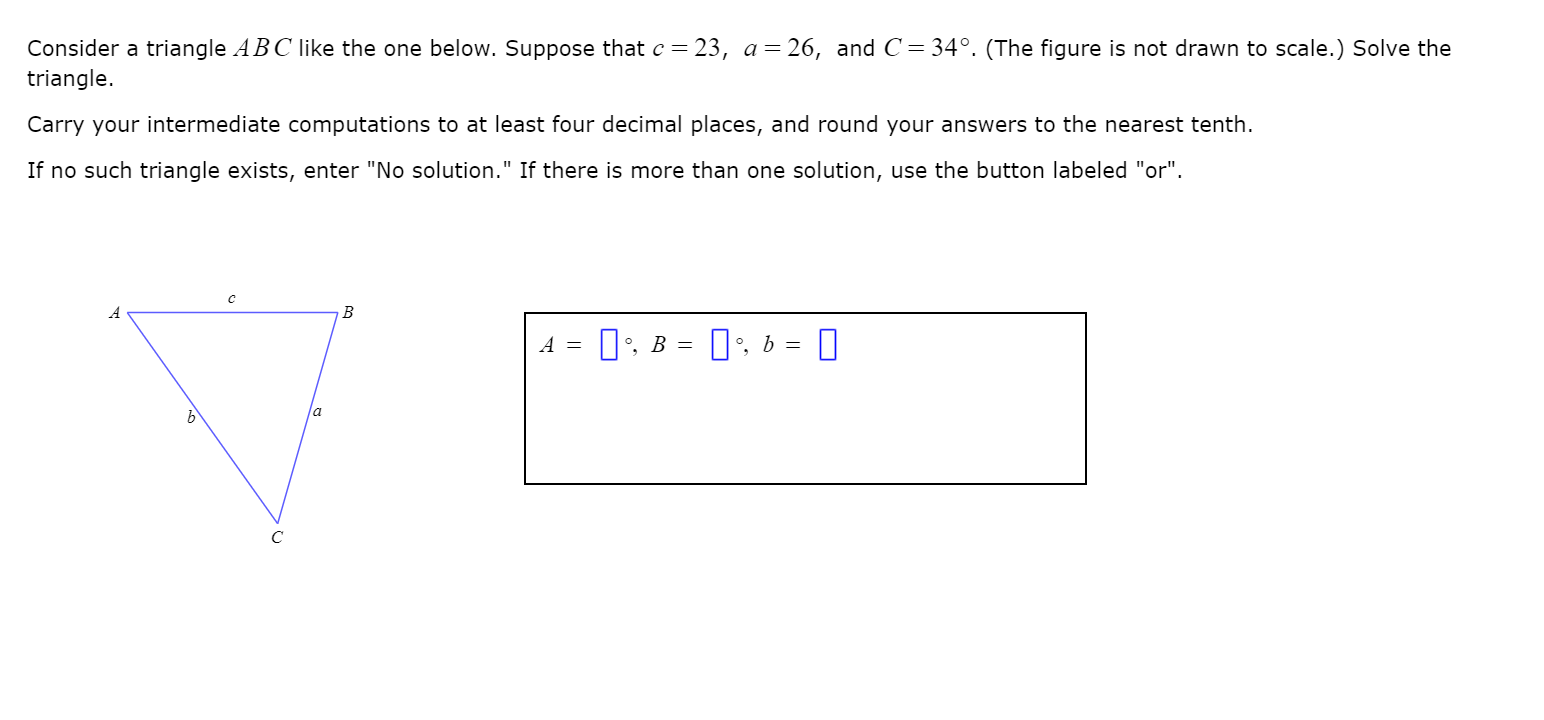# Consider a triangle ABC like the one below. Suppose that c = 23, a= 26, and C= 34°. (The figure is not drawn to scale.) Solve thetriangle.Carry your intermediate computations to at least four decimal places, and round your answers to the nearest tenth.If no such triangle exists, enter "No solution." If there is more than one solution, use the button labeled "or".0, 6 =|', B =la

Question
4 viewshelp_outlineImage TranscriptioncloseConsider a triangle ABC like the one below. Suppose that c = 23, a= 26, and C= 34°. (The figure is not drawn to scale.) Solve the triangle. Carry your intermediate computations to at least four decimal places, and round your answers to the nearest tenth. If no such triangle exists, enter "No solution." If there is more than one solution, use the button labeled "or". 0, 6 = |', B = la fullscreen
check_circle

Step 1

Given:

c = 23, a = 26 and C = 34°

To find:

Step 2

Concept:

Cosine formula:

Cosine formula given the relation between one angle and the three sides of the oblique triangle.

Step 3

For the value of sid...

### Want to see the full answer?

See Solution

#### Want to see this answer and more?

Solutions are written by subject experts who are available 24/7. Questions are typically answered within 1 hour.*

See Solution
*Response times may vary by subject and question.
Tagged in

### Trigonometry